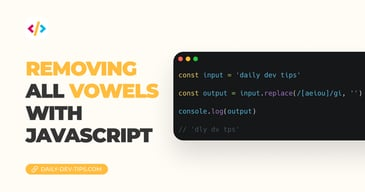Subscribe

# Vanilla JavaScript == vs ===

••••••• + 12 devs liked it

✍️

What exactly is the difference between == and ===?

8 Jun, 2020 · 2 min read

The difference between two or three = signs in `JavaScript` can be complicated and vague to understand at first. I found myself in a spot where I would always use the triple === set up to be safe. But let's try and find out the real difference today.

## JavaScript == vs ==== permalink

Let's first try and understand the basic difference on paper. The two are comparison operators, meaning they will compare between two sides; these can be variables or values.

The == operator is used for `equal to` comparison and is the most basic of the two.

Some options are:

``````x = 3;
console.log(x == 10); // false
console.log(x == 3); // true
console.log(x == '3'); // true``````

As you can see, we define a number, but even the string is valid.

The === comparison is the same, but stricter, it needs an equal value and equal type.

So doing the same we will get the following results:

``````x = 3;
console.log(x === 10); // false
console.log(x === 3); // true
console.log(x === '3'); // false``````

Hopefully, this made the initial difference clear between the two. Let me know if you want to see more of this in a future article.

See it in action on this Codepen.

See the Pen Vanilla JavaScript == vs === by Chris Bongers (@rebelchris) on CodePen.

Tweet this tip
••••••• + 12 devs liked it

### Removing all vowels with JavaScript

3 Dec, 2022 · 2 min read### 10 games to learn JavaScript

2 Dec, 2022 · 3 min readJoin 2101 devs and subscribe to my newsletter

• 1000 articles written
• 2101 devs subscribed
• 529116 words written